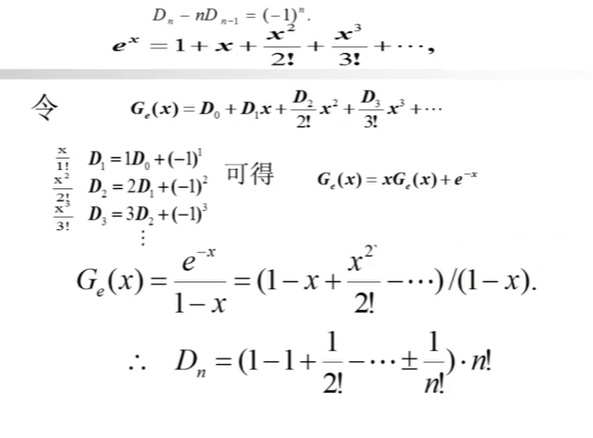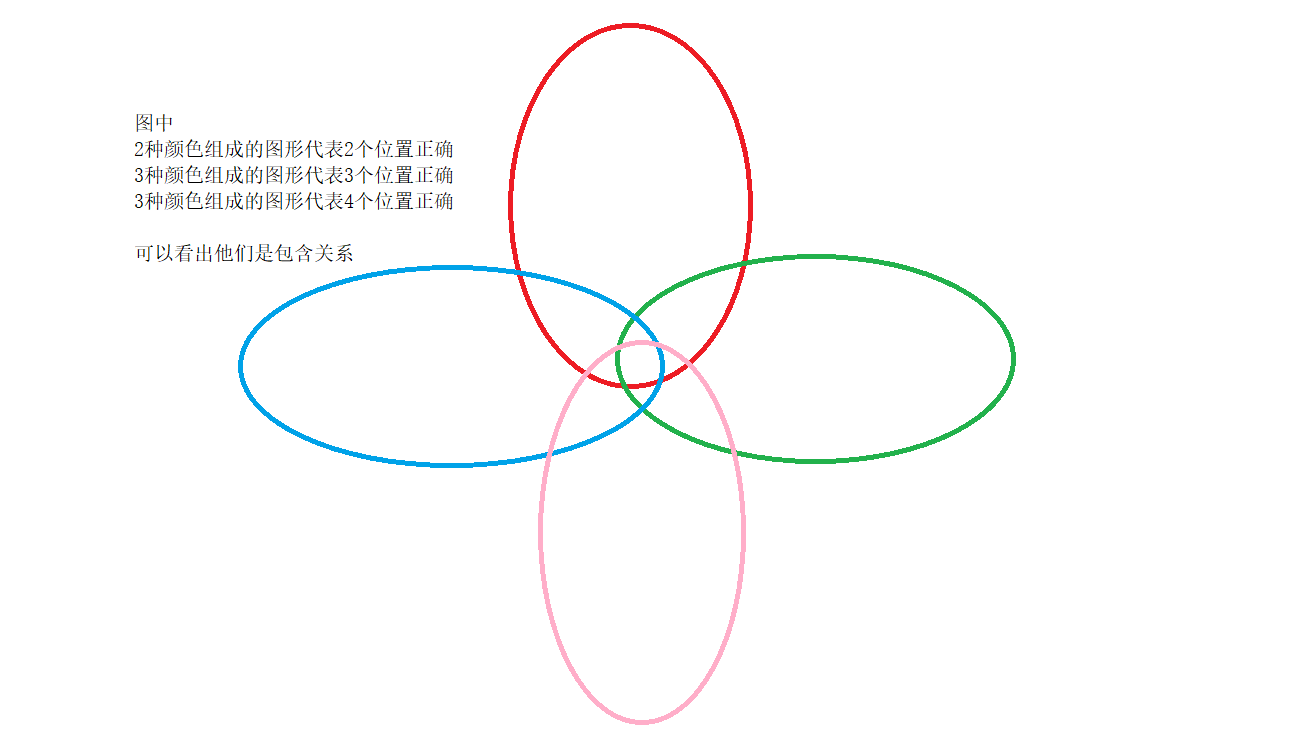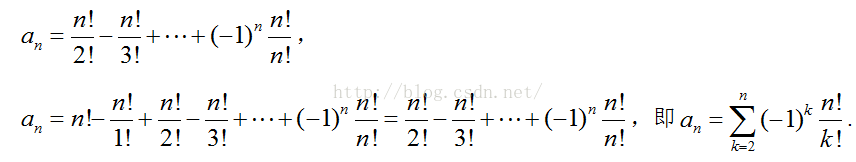• ## 错排

2020-09-18 14:21:58
// 一个元素没有错排 if(n==2)return 1; // 二个元素一种错排 return(n-1)*(f(n-1)+f(n-2)); //递推式 } 有n个数错排 ：第一个数有除一以外的(n-1)种，倘若它在第k个元素的位置上，对于第k个元素而言...
   int f (int n)  {
if(n==1)return 0;      // 一个元素没有错排
if(n==2)return 1;      // 二个元素一种错排
return(n-1)*(f(n-1)+f(n-2));    //递推式
}


有n个数错排     ：第一个数有除一以外的(n-1)种，倘若它在第k个元素的位置上，对于第k个元素而言，它所在的位置就有两种可能—第一种，它处在非第一个元素①位置上，接下来就相当于是n-1个元素的错排，即 f(n-1);
第二种，它处在第一个元素①的位置上，所以在排列D(n)中有两个元素找到了位置，那么接下来的队列就相当于是n-2个元素的错排。f(n-2)         最终递推式
f(n) =  (n-1)*[f(n-1)+f(n-2)]
法二：  通过组合数学母函数的求解括号内为    的泰勒展开，                   最接近 n！/e   的整数是错排个数


展开全文算法
• 关于错排的定义，可以自行百度 其中也有错排种数的计算 这个算法是基于全排列，在全排列添加条件。采用回溯，过程有点类似于剪支（那个条件的判断是否就不用继续执行了） import java.util.*; public class ...
关于错排的定义，可以自行百度 其中也有错排种数的计算
这个算法是基于全排列，在全排列添加条件。采用回溯，过程有点类似于剪支（那个条件的判断是否就不用继续执行了）
import java.util.*;

public class Derangements {
public List<List<Integer>> derangements(int[] nums) {
int len = nums.length;
// 使用一个动态数组保存所有可能的全排列
List<List<Integer>> res = new ArrayList<>();
if (len == 0) {
return res;
}

// 错排的定义是：n个有序的元素应有n！种不同的排列。如若一个排列式的所有的元素都不在原来的位置上，则称这个排列为错排

boolean[] used = new boolean[len];
Deque<Integer> path = new ArrayDeque<>(len);

dfs(nums, len, 0, path, used, res);
return res;
}

private void dfs(int[] nums, int len, int depth,
Deque<Integer> path, boolean[] used,
List<List<Integer>> res) {
if (depth == len) {
// System.out.println(Arrays.toString(path.toArray(path.toArray(new Integer))));
System.out.println(Arrays.toString(path.toArray()));
return;
}

for (int i = 0; i < len; i++) {
// 没有被使用，并且当前要使用的元素与当前位置不对应
if (!used[i] && i!=depth) {
used[i] = true;

// System.out.println("  递归之前 => " + path);
dfs(nums, len, depth + 1, path, used, res);

used[i] = false;
path.removeLast();
// System.out.println("递归之后 => " + path);
}
}
}
}


展开全文• 一、错排问题、 二、错排问题递推公式推导、 三、推导错排公式、


文章目录
一、错排问题二、错排问题递推公式推导三、推导错排公式

一、错排问题

n

n

封不同的信 与

n

n

个不同的信封 , 将

n

n

封信都装错信封的方案个数 ;

错排 ( Derangement ) , 因此错排公式中使用

D

(

n

)

D(n)

表示

n

n

个元素的错排 ;
假如有

1

1

封信 ,

n

=

1

n= 1

, 此时不能错排 ,

D

(

1

)

=

0

D(1) = 0

;
假如有

2

2

封信 ,

n

=

2

n= 2

, 此时交换一下即可完成错排, 有

1

1

种方案 ,

D

(

2

)

=

1

D(2) = 1

;假如有

3

3

封信 ,

1

,

2

,

3

1,2,3

三封信与三个信封 ,
如果

1

1

放到

3

3

信封中 , 此时将

2

2

放到

1

1

信封中 , 将

3

3

放到

2

2

信封中 ,

1

,

2

,

3

1,2,3

3

,

1

,

2

3,1,2

如果

1

1

放到

2

2

信封中 , 此时将

2

2

放到

3

3

信封中 , 将

3

3

放到

1

1

信封中 ,

1

,

2

,

3

1,2,3

2

,

3

,

1

2,3,1

D

(

3

)

=

2

D(3) = 2

如果有

4

4

封信 , 此时就不好计算 ,

9

9

种方法 ,

D

(

4

)

=

9

D(4) = 9

如果有

5

5

封信

⋯

\cdots

,

D

(

5

)

=

44

D(5) = 44

⋮

\vdots

如果有

n

n

封信

⋯

\cdots

,

D

(

n

)

=

n

!

(

(

−

1

)

0

0

!

+

(

−

1

)

1

1

!

+

(

−

1

)

2

2

!

+

(

−

1

)

3

3

!

+

⋯

+

(

−

1

)

n

n

!

)

=

n

!

∑

k

=

0

n

(

−

1

)

k

k

!

D(n) = n! ( \cfrac{(-1)^0}{0!} + \cfrac{(-1)^1}{1!} + \cfrac{(-1)^2}{2!} + \cfrac{(-1)^3}{3!} + \cdots + \cfrac{(-1)^n}{n!} ) = n! \sum\limits_{k=0}^{n}\cfrac{(-1)^k}{k!}

二、错排问题递推公式推导

观察上述规律 , 推导出递推公式 ;
假如有

n

n

封信 , 任何一封信都需要错位 , 错排方案数是

D

(

n

)

D(n)

;

1 . 分步计数原理 : 使用 分步计数原理 , 先统计 第一封信的排列方法 , 然后再讨论 其余信的排列方法数 ;
( 1 ) 第一步 : 首先找出一封信

a

a

出来 , 这封信不能排在其本身位置 , 只能放在其余

n

−

1

n-1

个位置上 , 因此有

n

−

1

n-1

种排法 ;
( 2 ) 第二步 : 现在讨论其余除

a

a

之外的其余信的位置的错排问题 ;

2 . 分类计数原理
假设第一封信

a

a

占据了

b

b

的位置 , 那么此时

b

b

放在哪个信封分两种情况 ,

b

b

放在

a

a

位置 , 或

b

b

不放在

a

a

位置 ;

( 1 ) 第一类 : 第一种情况是放在

a

a

位置 , 此时

b

b

放在

a

a

位置 , 剩下

n

−

2

n-2

封信进行错排 , 方案数是

D

(

n

−

2

)

D(n-2)

( 2 ) 第二类 : 第二种情况是

b

b

没有去

a

a

的位置 , 那么

b

b

可能出现在除

a

a

之外的任何位置 ,

b

b

有

n

−

2

n-2

个位置可以去 , 不能去

a

,

b

a,b

位置 , 其余所有元素都有

n

−

2

n-2

个位置可以去 (

a

,

b

a,b

位置不能去 ) , 这种情况下 相当于除

a

a

之外的其它元素的错排问题 , 即

n

−

1

n-1

个元素的错排问题 , 方案数是

D

(

n

−

1

)

D(n-1)

; ★ ( 核心推导逻辑 ) ★
( 3 ) 加法法则 : 汇总上述分类计数原理 , 使用 加法法则 , 计算结果是

D

(

n

−

1

)

+

D

(

n

−

2

)

D(n -1) + D(n-2)

3 . 乘法法则 : 汇总上述分步计数原理 , 使用 乘法法则 , 计算结果是

D

(

n

)

=

(

n

−

1

)

(

D

(

n

−

1

)

+

D

(

n

−

2

)

)

D(n) = (n-1) (D(n -1) + D(n-2))

三、推导错排公式

递推公式 :

D

(

n

)

=

(

n

−

1

)

(

D

(

n

−

1

)

+

D

(

n

−

2

)

)

D(n) = (n-1) (D(n -1) + D(n-2))

初值 :

D

(

1

)

=

0

,

D

(

2

)

=

1

D(1) = 0 , D(2) = 1

使用 递推方程 , 生成函数求解递推方程 , 容斥原理 , 可以推导出如下公式 :

D

(

n

)

=

n

!

(

(

−

1

)

0

0

!

+

(

−

1

)

1

1

!

+

(

−

1

)

2

2

!

+

(

−

1

)

3

3

!

+

⋯

+

(

−

1

)

n

n

!

)

=

n

!

∑

k

=

0

n

(

−

1

)

k

k

!

D(n) = n! ( \cfrac{(-1)^0}{0!} + \cfrac{(-1)^1}{1!} + \cfrac{(-1)^2}{2!} + \cfrac{(-1)^3}{3!} + \cdots + \cfrac{(-1)^n}{n!} ) = n! \sum\limits_{k=0}^{n}\cfrac{(-1)^k}{k!}

参考 :
百度百科-错排公式【组合数学】递推方程 ( 递推方程求解过程总结 | 齐次 | 重根 | 非齐次 | 特征根为 1 | 指数形式 | 底为特征根的指数形式 ) ★★【组合数学】生成函数 ( 生成函数应用场景 | 使用生成函数求解递推方程 )【集合论】容斥原理 ( 包含排斥原理 | 示例 )
展开全文• 视第一步的结果，若1号元素落在第 k 个位置，第二步就先把 k 号元素“错排”好， k 号元素的不同排法将导致两类不同的情况发生： ① k 号元素排在第1个位置，留下的 n - 2 个元素在与它们的编号集相等的位置集上...

题目：年会分奖 描述：今年公司年会的奖品特别给力，但获奖的规矩却很奇葩：
首先，所有人员都将一张写有自己名字的字条放入抽奖箱中；待所有字条加入完毕，每人从箱中取一个字条；如果抽到的字条上写的就是自己的名字，那么“恭喜你，中奖了！”
现在告诉你参加晚会的人数，请你计算有多少概率会出现无人获奖？ 输入描述: 输入包含多组数据，每组数据包含一个正整数n（2≤n≤20）。 输出描述: 对应每一组数据，以“xx.xx%”的格式输出发生无人获奖的概率。 示例1 输入 2 输出 50.00%

这是牛客网上一道典型的错排算法题。
错排算法，简单来说就是n个元素，让他们重新排列后都不在自己的位置上。设有n个元素，共有m种错排方式
n = 1, 那么 m = 0;n = 2, 有[2,1]一种排列方式 ， m = 1；n = 3， 有[2, 3, 1], [3, 1, 2]两种， m = 2；n = 4， m = 9，具体可以自己枚举。
可以看出，n越大，需要枚举的次数就越多，这时就需要找出通解。
我们拿n = 4举例： ①全排列共 4！，由于 全排列次数 = 错排 + 正确。所以我们需要排除存在正确位置的排列项即可，而1排正确的次数有 3！，2排正确的次数有3！。 所以错排次数 = 4！- 4 × 3! ②那么此时存在一个问题，在减去排一次正确的时候多减了排两次正确的次数，比如1, 2排正确有[1, 2, 4, 3], 2, 1排正确有[1, 2, 4,3],就减了两次。此时需要加上排两次正确的次数。两个正确的组合有6种(12,13,14,23,24,34)，剩余两个随便排2！ 所以错排次数 = 4！- 4 × 3 ! + 6 × 2. ③又存在一个问题，在加上两个正确的组合后却多加了三个正确的组合，比如，1，2, 3位置正确时我们加了[1,2,3,4]这个组合，那么在2，3, 1位置正确的时候，我们也加了[1,2,3,4]这个组合，所以此时应该减去3次正确的次数，三个正确的组合有（123，134，124，234）四种，剩余一个排就1种。 所以错排次数 = 4！- 4 × 3 ! + 6 × 2 - 4 × 1. ④又有问题了，在减去三个正确的组合时，多减了4个正确的组合，比如1，2，3， 4位置正确时减去了[1,2,3,4], 2,3,4,1位置正确时减去了[1,2,3,4].所以应该加上4个正确的情况，[1,2,3,4]. 所以错排次数 = 4！- 4 × 3 ! + 6 × 2 - 4 × 1 + 1 = 9. 下面这个图方便理解，将面积作为次数，次数加减相当于图形面积的加减，可以看出，每次涉及到图形加减时都会重复的加或减去多个图形公共的部分，就比如要算 红色和绿色的面积 = 红 + 绿 - 红绿相交。所以总结出来的公式为还有一个理解方法， 解释：n个不同的元素的错排。
1：首先我们先错排第1号元素，第一号元素可以排的位置有第二个位置到第n个位置，那么第一步有（n-1）种情况；
2：第二步，“错排”其余 n - 1 个元素，按如下顺序进行。视第一步的结果，若1号元素落在第 k 个位置，第二步就先把 k 号元素“错排”好， k 号元素的不同排法将导致两类不同的情况发生： ① k 号元素排在第1个位置，留下的 n - 2 个元素在与它们的编号集相等的位置集上“错排”，有 f(n -2) 种方法； ② k 号元素不排第 1 个位置，这时可将第 1 个位置“看成”第 k 个位置(也就是说本来准备放到k位置为元素，可以放到1位置中),于是形成（包括 k 号元素在内的） n - 1 个元素的“错排”，有 f(n - 1) 种方法。据加法原理，完成第二步共有 f(n - 2)+f(n - 1) 种方法。 根据乘法原理， n 个不同元素的错排种数 f(n) = (n-1)[f(n-2)+f(n-1)] (n>2) 。
展开全文• ## 错排公式详解

千次阅读 多人点赞 2019-07-19 23:21:58
在HDU刷题时遇到了关于错排公式的一些问题。本篇文章将详细解释错排公式的推导过程。 错排的定义：一段序列中一共有n个元素，那么可知这些元素一共有n!种排列方法。假如在进行排列时，原来所有的元素都不在原来的...小结 HDU
• 文章目录错排问题例题引入例题1代码例题2代码 错排问题 n个有序的元素应有n!个不同的排列，如若一个排列使得所有的元素不在原来的位置上，则称这个排列为错排；有的叫重排。——百度百科 嗯，这就是错排 例题引入 ...
• 错排问题，是组合数学中的问题之一。考虑一个有n个元素的排列，若一个排列中所有的元素都不在自己原来的位置上，那么这样的排列就称为原排列的一个错排。 n个元素的错排数记为D(n)。研究一个排列错排个数的问题，...算法
• 文章目录递推关系容斥原理棋盘多项式莫比乌斯反演 考虑这么一个问题：????个元素依次给以标号????，?...个元素的全排列 中，每个元素都不在自己原来位置上的排列数。...个数之一互换，其余n-2个数进 行错排，共得(???? −
• ## 错排问题--错排公式的推导及应用

万次阅读 多人点赞 2017-08-12 11:05:19
错排问题是组合数学中的问题之一。考虑一个有n个元素的排列，若一个排列中所有的元素都不在自己原来的位置上，那么这样的排列就称为原排列的一个错排。 n个元素的错排数记为Dn。 研究一个排列错排个数的问题，...应用 数学
• 个不同的排列，如若一个排列使得所有的元素不在原来的位置上，则称这个排列为错排；有的叫重排。 如，1 2的错排是唯一的，即2 1。1 2 3的错排有31 2，2 3 1。这二者可以看作是1 2错排，3分别与1、2换位而得的。 错排...算法
• 错排问题 n个有序的元素应有n!个不同的排列，如若一个排列使得所有的元素不在原来的位置上，则称这个排列为错排；有的叫重排。 错排公式 递推关系 为求其递推关系，分两步走： 第一步，考虑第n个元素，把它放在某一...
• ## 错排问题(全错排)

千次阅读 2018-11-11 17:15:37
错排问题(全错位排列问题 Derangement) 概念： 考虑一个有n个元素的排列，若一个排列中所有的元素都不在自己原来的位置上，那么这样的排列就称为原排列的一个错排。 n个元素的错排数记为D(n)。 研究一个排列错排个...
• 考虑一个有n个元素的排列，若一个排列中所有的元素都不在自己原来的位置上，那么这样的排列就称为原排列的一个错排。 n个元素的错排数记为D(n)。 研究一个排列错排个数的问题，叫做错排问题或称为更列问题。 典型...
• 错排问题是组合数学中的问题之一。考虑一个有n个元素的排列，若一个排列中所有的元素都不在自己原来的位置上，那么这样的排列就称为原排列的一个错排。 n个元素的错排数记为Dn。 研究一个排列错排个数的问题，叫做...
• f[n] 表示从第一个放到第n个的最大错排方案数，则 假设咱们要放第n号物品，首先把第n号物品拿在手里，那么n号就有一个空位了，现在咱们把第n号物品随意放在一个位置，因为是错排，不能放在n上，所以有(n - 1)个位置......